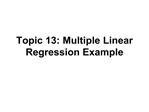# Topic 13: Multiple Linear Regression Example - PowerPoint PPT PresentationDownload PresentationTopic 13: Multiple Linear Regression Example

Topic 13: Multiple Linear Regression Example
Download Presentation## Topic 13: Multiple Linear Regression Example

- - - - - - - - - - - - - - - - - - - - - - - - - - - E N D - - - - - - - - - - - - - - - - - - - - - - - - - - -
##### Presentation Transcript

1. Topic 13: Multiple Linear Regression Example

3. Study of CS students Computer science majors at Purdue have a large drop out rate Can we find predictors of success Predictors must be available at time of entry into program

4. Data available GPA after three semesters High school math grades High school science grades High school English grades SAT Math SAT Verbal Gender (of interest for other reasons)

5. Data for CS Example Y is grade point average 3 HS grades and 2 SATs are the explanatory variables (p=6) Have n=224 students

6. Descriptive Statistics

7. Output from Proc Means

8. Output from Proc Means

9. Descriptive Statistics

11. High School Math

12. High School Science

13. High School English

14. SAT Math

15. SAT Verbal

16. Interactive Data Analysis Click on menu Solutions -> analysis -> interactive data analysis Obtain SAS/Insight window Open library work Click on Data Set A1 (if it exists) Open

17. Scatter Plot Matrix (shift) Click on GPA, SATM, SATV Go to menu Analyze Choose option Scatterplot(XY) Try some other options

18. Scatter Plot Matrix

19. Correlations

20. Output from Proc Corr

21. Output from Proc Corr

22. Output from Proc Corr

23. Use High School Grades to predict GPA

25. CS ANOVA Table

26. Remove HSS

28. Rerun with HSM only

30. SATs

32. HS and SATs

36. Best Model?

37. Key ideas from case study First, look at graphical and numerical summaries for one variable at a time Then, look at relationships between pairs of variables with graphical and numerical summaries. Use plots and correlations

38. Key ideas from case study The relationship between a response variable and an explanatory variable depends on what other explanatory variables are in the model A variable can be a significant (P<.05) predictor alone and not significant (P>0.5) when other X�s are in the model

39. Key ideas from case study Regression coefficients, standard errors and the results of significance tests depend on what other explanatory variables are in the model

40. Key ideas from case study Significance tests (P values) do not tell the whole story Squared multiple correlations give the proportion of variation in the response variable explained by the explanatory variables) can give a different view We often express R2 as a percent

41. Key ideas from case study You can fully understand the theory in terms of Y = X� + ? To effectively use this methodology in practice you need to understand how the data were collected, the nature of the variables, and how they relate to each other